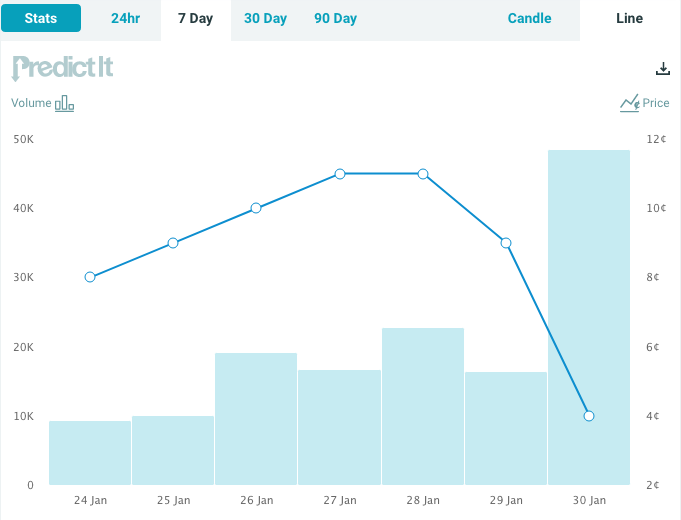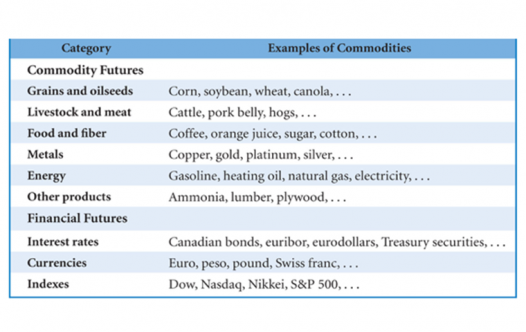# On the role of replicating portfolios in the pricing of financial derivatives in general

Replicating portfolios play a central role in terms of pricing financial derivatives. Here is what we have learned so far about replicating portfolios in Finance 4366:

1. Replicating portfolios for long and short forward contracts. Buying forward is equivalent to buying the underlying on margin, and selling forward is equivalent to shorting the underlying and lending money. Like options, forwards and futures are priced by pricing the replicating portfolio and invoking the “no-arbitrage” condition. If the forward/futures price it too low, then one can earn positive returns with zero risk and zero net investment by buying forward, shorting the underlying and lending money. Similarly, if the forward futures price is too high, one can earn positive returns with zero risk and zero net investment by selling forward and buying the underlying with borrowed money. This is commonly referred to as “riskless arbitrage”; it’s riskless because you’re perfectly hedged, and it’s arbitrage because you are buying low and selling high.
2. Replicating portfolio for a call option. The replicating portfolio for a call option is a margined investment in the underlying. For example, in my teaching note entitled “A Simple Model of a Financial Market”, I provide a numerical example where the interest rate is zero, there are two states of the world, a bond which pays off \$1 in both states is worth \$1 today, and a stock that pays off \$2 in one state and \$.50 in the other state is also worth one dollar. In that example, the replicating portfolio for a European call option with an exercise price of \$1 consists of 2/3 of 1 share of stock (costing \$0.66) and a margin balance consisting of a short position in 1/3 of a bond (which is worth -\$0.33). Thus, the arbitrage-free value of the call option is \$0.66 – \$0.33 = \$0.33.
3. Replicating portfolio for a put option. Since the replicating portfolio for a call option is a margined investment in the underlying, it should come as no surprise that the replicating portfolio for a put option consists of a short position in the underlying combined with lending. Thus, in order to price the put, you need to determine and price the components of the replicating portfolio.  Continuing with the same numerical example described for the call option in part 2 above,  the replicating portfolio for an otherwise identical European put option with an exercise price of \$1 consists of a short position in 1/3 of 1 share of stock (which is worth -\$0.33), combined with a long position in 2/3 of a bond (which is worth \$0.66). Thus, the arbitrage-free value of the put option is  – \$0.33 + \$0.66 = \$0.33.
4. Put-Call Parity. Note also that if you know the value of a call, the underlying, and the present value of the exercise price, then you can apply the put-call parity equation to figure out the price for the put option; i.e.,${C_0} + PV(K) = {P_0} + {S_0} \Rightarrow {P_0} = {C_0} + PV(K) - {S_0}.$ Since we know the price of the call (\$0.33), the present value of the exercise price (\$1), and the stock price (\$1), then it follows from the put-call parity equation that the value of the put is also 33 cents. More generally, if you know the values of three of the four securities that are included in the put-call parity equation, then you can infer the “no-arbitrage” value of the fourth security.

# Problem Set 3, due Thursday, 2/6, is now available

I just posted Problem Set 3 on the course website. It is due at the beginning of class on Thursday, 2/6, and it is based on material that we will cover during tomorrow’s class meeting.

# Mark your calendars – Finance 4366 extra credit opportunity!

I have decided to offer the following extra credit opportunity for Finance 4366. You can earn extra credit by attending and reporting on Dr. Michael Rectenwald’s upcoming talk entitled “Woke Capitalism: Qui Bono?” Dr. Rectenwald’s talk is scheduled for Thursday, February 13 from 5:15-6:30 p.m. in Foster 250.

If you decide to take advantage of this opportunity, I will use the grade you earn on your report to replace your lowest quiz grade in Finance 4366 (assuming that your grade on the extra credit is higher than your lowest quiz grade). The report should be in the form of a 1-2 page executive summary in which you provide a critical analysis of Dr. Rectenwald’s lecture. In order to receive credit, the report must be submitted via email to fin4335@gmail.com in either Word or PDF format by no later than Monday, February 17 at 5 p.m.# The latest prediction market assessment

In a blog post last week entitled “Prediction markets’ take on removal of POTUS from office“, I wrote about the PredictIt.org prediction market, where one could purchase a “share” which pays \$1 if the answer to the question, “Will the Senate convict Donald Trump in his first term?, turns out to be “yes”. Since the price of that share at that time was 8 cents, this meant that investors at the time were placing an 8% probability that POTUS would be removed from office as a consequence of the Senate trial.

Since last week, the daily closing prices of the share price have fluctuated between a high of 11 cents (on 1/27 and 1/28) and a low of 4 cents (on 1/30); see the graph below:It will be interesting to see how the share price changes going forward. Given the political composition of the US Senate, it is not surprising that the odds of conviction are as low as they are, but expect more share price volatility as this political drama plays out. After all, the only two possibilities are for the share price to go to either \$0 or \$1.

# Examples of commodity and financial futures contracts traded on U.S. exchangesFor a non-technical introduction to forward and futures contracts, it’s hard to beat the following video tutorial on this topic:

# Finance 4366 Grades on Canvas

I have posted Finance 4366 numeric course grades to Canvas; the FIN 4366 grade book is at https://baylor.instructure.com/courses/108333/gradebook. To date, we have had five class meetings, three quizzes (two of which have been graded), and three problem sets (problem sets 1, 2, and the student questionnaire which was graded on a (0, 100) basis; the grade for problem set 2 is still pending). Since we haven’t had any exams yet, the course grade which now appears on Canvas was calculated using the following equation:

Current Course Numeric Grade = (.10(Class Attendance) + .10(Quizzes) + .20(Problem Sets))/.4

Or course, this is simply a special case of the final course numeric grade equation given in the course syllabus:

Final Course Numeric Grade = .10(Class Attendance) + .10(Quizzes) + .20(Problem Sets) + Max{.20(Midterm Exam 1) + .20(Midterm Exam 2) + .20(Final Exam), .20(Midterm Exam 1) + .40(Final Exam), .20(Midterm Exam 2) + .40(Final Exam)}

As time passes and I continue to collect grade data from you, the grades as reported on Canvas will be posted on a timely basis. Once Midterm 1 grades are determined, then the Canvas course grade will be calculated using the following equation:

Course Numeric Grade after Midterm 1 = (.10(Class Attendance) + .10(Quizzes) + .20(Problem Sets) + .20(Midterm 1))/.6

Once Midterm 2 grades are determined, then the following equation will be used:

Course Numeric Grade after Midterm 2 = (.10(Class Attendance) + .10(Quizzes) + .20(Problem Sets) + .20(Midterm 1) + .20(Midterm 2))/.8

After I record final exam grades, I will use the Final Course Numeric Grade equation above to determine your final course numeric grade, and (as also noted in the course syllabus), the final course letter grade will be based upon the following schedule of final course numeric grades:

 A 93-100% C 73-77% A- 90-93% C- 70-73% B+ 87-90% D+ 67-70% B 83-87% D 63-67% B- 80-83% D- 60-63% C+ 77-80% F <60%

# Problem Set 2 question

Here’s a brief Q&A that I had last evening with a student about Problem Set 2:

Q: Hi Dr. Garven, I was wondering if I should use =stdev.p or =stdev.s when solving for the standard deviation on Excel. I understand this is a sample so =stdev.s is the formula I presume that I should use but I’m not quite sure. Which should I use?

A: Neither – the “=stdev.p” and “=stdev.s” commands in Excel are useful for calculating the standard deviation of observed realized values of a random variable. In problem set 2, the investor contemplates expectations of future state-contingent returns on securities. Since standard deviation is the square root of the variance, start by calculating variance (see p. 9 of the Statistics Tutorial, Part 1 lecture note).